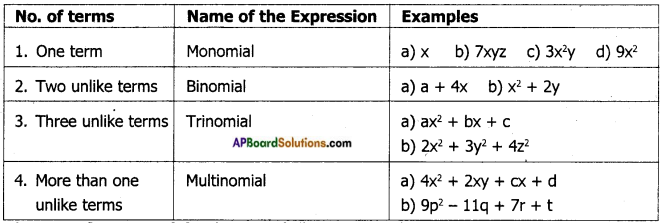Students can go through AP Board 7th Class Maths Notes Chapter 10 Algebraic Expressions to understand and remember the concepts easily.

## AP State Board Syllabus 7th Class Maths Notes Chapter 10 Algebraic Expressions

→ Variable: It takes different values.
Ex: x, y, z, a, b, c, m etc.
Constant: The value of constant is fixed.
Ex: 1, 2, $$\frac{-2}{3}$$, $$\frac{4}{5}$$ etc.

→ Algebraic Expression: An algebraic expression is a single term or a combination of terms connected by the symbols ‘+’ (plus) or(minus).
Ex: 2x + 3, $$\frac{2}{5}$$p, 3x – 1 etc.→ Numerical Expression : If every term of an expression is a constant term, then the expression is called a Numerical expression.
Ex: 2 + 1, -5 × 3, (12 + 4) ÷ 3.
Note: In the expression 2x + 9, ‘2x’ is an algebraic term. ‘9’ is called numeric term.

→ Like terms are terms which contain the same variables with the same exponents.
Ex: 12x, 25x, -7x are like terms.
2xy2, 3xy2, 7xy2 are like terms.

→ Coefficient: In a.xn, ‘a’ is called the numerical coefficient and ‘x’ is called the literal coefficient.
Types of algebraic expressions.→ Degree of a monomial: The sum of all exponents of the variables present in a monomial is called the degree of the term or degree of the monomial.
Ex: The degree of 9x2y2 is 4 [∵ 2 + 2 = 4]
Note: Degree of constant term is zero.
The highest of the degrees of all the terms of an expression is called the degree of the expression.
Ex: The degree of the expression ax + bx2 + cx3 + dx4 + ex5 is 5.→ The difference between two like terms is a like term with a numerical coefficient equal to the difference between the numerical coefficients of the two like terms.
Note:

1. If no two terms of an expression are alike then it is said to be in the simplified form.
2. In an expression, if the terms are arranged in such a way that the degrees of the terms are in descending order then the expression is said to be in standard form.
3. Addition (or) subtraction of expressions should be done in two methods, they are
i) Column or Vertical method.
ii) Row or Horizontal method.